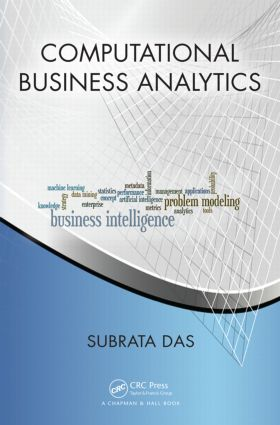## 1st Edition

Chapman and Hall/CRC

516 pages | 290 B/W Illus.

Hardback: 9781439890707
pub: 2013-12-14
SAVE ~\$18.19
\$90.95
\$72.76
x
eBook (VitalSource) : 9780429066344
pub: 2013-12-14
from \$45.48

FREE Standard Shipping!

### Description

Learn How to Properly Use the Latest Analytics Approaches in Your Organization

Computational Business Analytics presents tools and techniques for descriptive, predictive, and prescriptive analytics applicable across multiple domains. Through many examples and challenging case studies from a variety of fields, practitioners easily see the connections to their own problems and can then formulate their own solution strategies.

The book first covers core descriptive and inferential statistics for analytics. The author then enhances numerical statistical techniques with symbolic artificial intelligence (AI) and machine learning (ML) techniques for richer predictive and prescriptive analytics. With a special emphasis on methods that handle time and textual data, the text:

• Enriches principal component and factor analyses with subspace methods, such as latent semantic analyses
• Combines regression analyses with probabilistic graphical modeling, such as Bayesian networks
• Extends autoregression and survival analysis techniques with the Kalman filter, hidden Markov models, and dynamic Bayesian networks
• Embeds decision trees within influence diagrams
• Augments nearest-neighbor and k-means clustering techniques with support vector machines and neural networks

These approaches are not replacements of traditional statistics-based analytics; rather, in most cases, a generalized technique can be reduced to the underlying traditional base technique under very restrictive conditions. The book shows how these enriched techniques offer efficient solutions in areas, including customer segmentation, churn prediction, credit risk assessment, fraud detection, and advertising campaigns.

Analytics Background and Architectures

ANALYTICS DEFINED

ANALYTICS MODELING

ANALYTICS PROCESSES

ANALYTICS AND DATA FUSION

Mathematical and Statistical Preliminaries

STATISTICS AND PROBABILITY THEORY

LINEAR ALGEBRA FUNDAMENTALS

MATHEMATICAL LOGIC

GRAPHS AND TREES

MEASURES OF PERFORMANCE

ALGORITHMIC COMPLEXITY

Statistics for Descriptive Analytics

PROBABILITY DISTRIBUTIONS

DISCRETE PROBABILITY DISTRIBUTIONS

CONTINUOUS PROBABILITY DISTRIBUTIONS

GOODNESS-OF-FIT TEST

Bayesian Probability and Inference

BAYESIAN INFERENCE

PRIOR PROBABILITIES

Inferential Statistics and Predictive Analytics

CHISQUARE

TEST OF INDEPENDENCE

REGRESSION ANALYSES

BAYESIAN LINEAR REGRESSION

PRINCIPAL COMPONENT AND FACTOR ANALYSES

SURVIVAL ANALYSIS

AUTOREGRESSION MODELS

Artificial Intelligence for Symbolic Analytics

ANALYTICS AND UNCERTAINTIES

NEO-LOGICIST APPROACH

NEO-PROBABILIST

NEO-CALCULIST APPROACH

NEO-GRANULARIST

Probabilistic Graphical Modeling

NAIVE BAYESIAN CLASSIFIER (NBC)

KDEPENDENCE NAIVE BAYESIAN CLASSIFIER (KNBC)

BAYESIAN BELIEF NETWORKS

Decision Support and Prescriptive Analytics

EXPECTED UTILITY THEORY AND DECISION TREES

INFLUENCE DIAGRAMS FOR DECISION SUPPORT

SYMBOLIC ARGUMENTATION FOR DECISION SUPPORT

Time Series Modeling and Forecasting

PROBLEM MODELING

KALMAN FILTER (KF)

MARKOV MODELS

DYNAMIC BAYESIAN NETWORKS (DBNS)

Monte Carlo Simulation

MONTE CARLO APPROXIMATION

GIBBS SAMPLING

METROPOLIS-HASTINGS ALGORITHM

PARTICLE FILTER (PF)

Cluster Analysis and Segmentation

HIERARCHICAL CLUSTERING

K-MEANS CLUSTERING

K-NEAREST NEIGHBORS

SUPPORT VECTOR MACHINES

NEURAL NETWORKS

Machine Learning for Analytics Models

DECISION TREES

LEARNING NAIVE BAYESIAN CLASSIFIERS

LEARNING OF KNBC

BAYESIAN BELIEF NETWORKS

INDUCTIVE LOGIC PROGRAMMING

Unstructured Data and Text Analytics

INFORMATION STRUCTURING AND EXTRACTION

BRIEF INTRODUCTION TO NLP

TEXT CLASSIFICATION AND TOPIC EXTRACTION

Semantic Web

RESOURCE DESCRIPTION FRAMEWORK (RDF)

DESCRIPTION LOGICS

Analytics Tools

INTELLIGENT DECISION AIDING SYSTEM (IDAS)

ENVIRONMENT FOR FIFTH GENERATION APPLICATIONS (E5)

ANALYSIS OF TEXT (ATEXT)

R AND MATLAB

SAS AND WEKA

Analytics Case Studies

RISK ASSESSMENT MODEL I3

RISK ASSESSMENT IN INDIVIDUAL LENDING USING IDAS

RISK ASSESSMENT IN COMMERCIAL LENDING USING E5 AND IDAS

FRAUD DETECTION

SENTIMENT ANALYSIS USING ATEXT

Appendix A: Usage of Symbols

Appendix B: Examples and Sample Data

Appendix C: MATLAB and R Code Examples

Index

Further Reading appears at the end of each chapter.

Subrata Das is the founder and president of Machine Analytics and also serves as a consulting scientist to other companies. He has many years of experience in industrial, government, and academic research and development. He earned his Ph.D. in computer science and master's in mathematics.